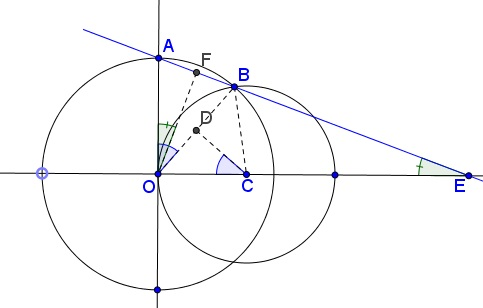# Two Circles and a Limit Proof #2 by Stuart Anderson

We are solving the Two Circles and a Limit problem:

A stationary circle of radius $3$ is centered at $(3, 0).$ Another circle of variable radius $r$ is centered at the origin and meets the positive $y-axis$ in point $A.$ Let $B$ be the common point of the two circles in the upper half-plane. Let $E$ be the intersection of $AB,$ extended, with the $x-axis.$ What happens to $E$ as $r$ grows smaller and smaller?

Let $C$ be the center of the stationary circle. Draw the chord $OB$ of the varying circle and consider the triangle $OCB.$ This is of course isosceles, so the angle bisector at $C$ cuts the chord $OB$ at its midpoint $D,$ and meets $OB$ at a right angle. Therefore $\angle AOB = \angle DCO = 1/2 \angle BCO.$ Next, look at triangle $AOB,$ which is also isosceles, and repeat the same process, so that there is an angle bisector at $O$ meeting chord $AB$ perpendicularly at its midpoint $F.$ Then since $OF$ is perpendicular to $BE,$ $\angle BEO = \angle AOF = 1/2 \angle AOB.$Therefore $\angle BEO = 1/4 \angle BCO.$ This is true regardless of the size of $r,$ so in the limit as $r→0,$ B moves down to the origin and in the small angle limit we get $OE = 4·OC.$ But $OC = 3,$ so $OE\to 12.$ One could put more details into taking this limit, but it is pretty clear that the ratio approaches $4.$ Although the algebraic methods also give the limit as $4,$ this appears somewhat mysterious. The fact that the angles $BCO$ and $BEO$ are in $4:1$ ratio gives the clearest most satisfying way to see why the limit is $4,$ at least in my opinion.### Limits in Geometry